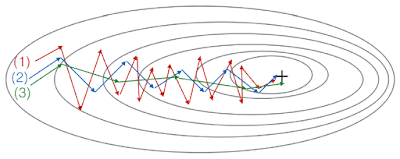# These plots were generated with gradient descent; with gradient descent with momentum (β = 0.5) and gradient descent with momentum (β = 0.9). Which curve corresponds to which algorithm?

1. Consider this figure:These plots were generated with gradient descent; with gradient descent with momentum (β = 0.5) and gradient descent with momentum (β = 0.9). Which curve corresponds to which algorithm?
•  (1) is gradient descent with momentum (small β), (2) is gradient descent with momentum (small β), (3) is gradient descent
•  (1) is gradient descent. (2) is gradient descent with momentum (large β) . (3) is gradient descent with momentum (small β)
•  (1) is gradient descent with momentum (small β). (2) is gradient descent. (3) is gradient descent with momentum (large β)
•  (1) is gradient descent. (2) is gradient descent with momentum (small β). (3) is gradient descent with momentum (large β)